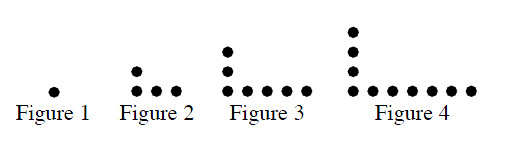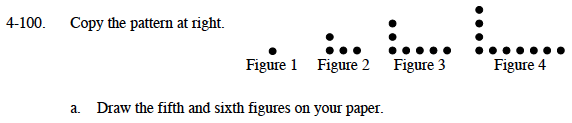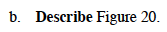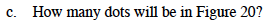### Home > MC1 > Chapter 4 > Lesson 4.3.2 > Problem4-100

4-100.
1. . Copy the pattern below. Homework Help ✎1. Draw the fifth and sixth figures on your paper.

2. Describe Figure 20.

3. How many dots will be in Figure 20?Refer to problem 3-32 if you are having trouble getting started with this problem.How would you describe Figure 1? Figure 2? Find a pattern so that you can imagine how Figure 20 will look, and then describe it in the same way you would Figures 1 and 2.

20 vertical dots and 39 horizontal dotsThe total number of dots in Figure 20 will be the sum of the vertical dots and the horizontal dots minus one (so that you do not count the corner dot twice).